# Find Angles Using Knowledge of Sectors

In this worksheet, students will use their knowledge of sectors to find angles when given an arc length and sector area.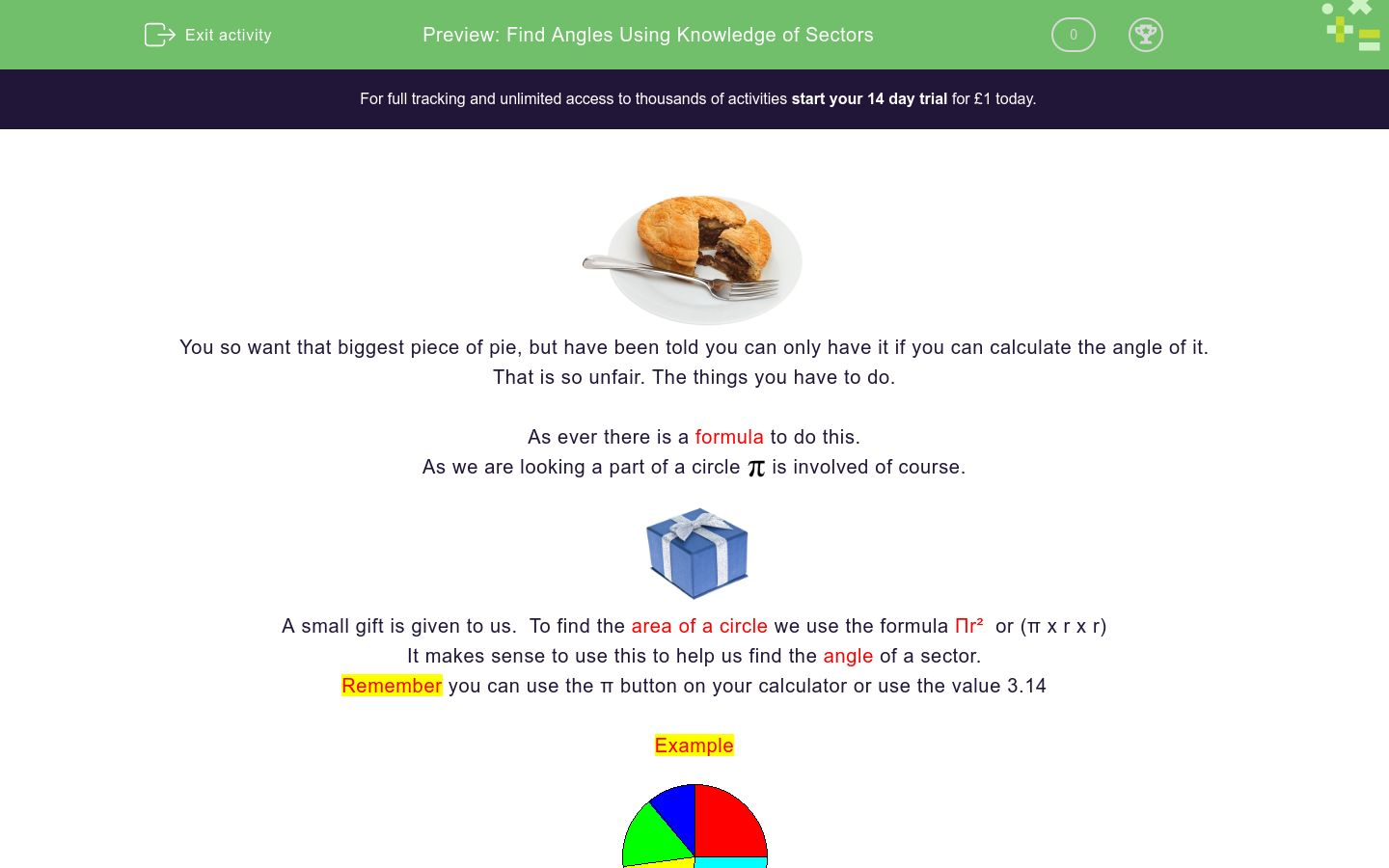Key stage:  KS 4

GCSE Subjects:   Maths

GCSE Boards:   AQA, OCR, Eduqas, Pearson Edexcel

Curriculum topic:   Geometry and Measures, Mensuration

Curriculum subtopic:   Mensuration and Calculation, Area Calculations

Difficulty level:### QUESTION 1 of 10You so want that biggest piece of pie, but have been told you can only have it if you can calculate the angle of it.

That is so unfair. The things you have to do.

As ever there is a formula to do this.

As we are looking a part of a circleis involved of course.A small gift is given to us.  To find the area of a circle we use the formula Πr²  or (π x r x r)

It makes sense to use this to help us find the angle of a sector.

Remember you can use the π button on your calculator or use the value 3.14

Example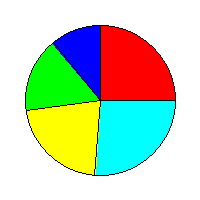The area of the dark blue sector of this pie chart is 25 cm²

The radius is 10 cm. Find the angle at the centre of this sector.

Angle = Area x 360 ÷ π x r x r

25 x 360 = 9000

3.14 x 10 x 10 = 314

9000 ÷ 314 = 28.7° correct to 1 decimal place

Just follow the steps of the formula carefully and you can't go wrong.

In the following activities 3.14 has been used as the value of πThe area of this slice of pie is 20 cm&sup2;

Find the angle at the centre of the pie.

Use 3.14 for the value of &Pi;

57.3°

86.0°

63.7°

72.2°The area of the light blue part of this pie chart is 75 cm²

Find the angle at the centre of that sector of the pie chart.

Use 3.14 for the value of Π

 86.0° 85.9° 83.2° 84.3° The angle at the centre of the sector is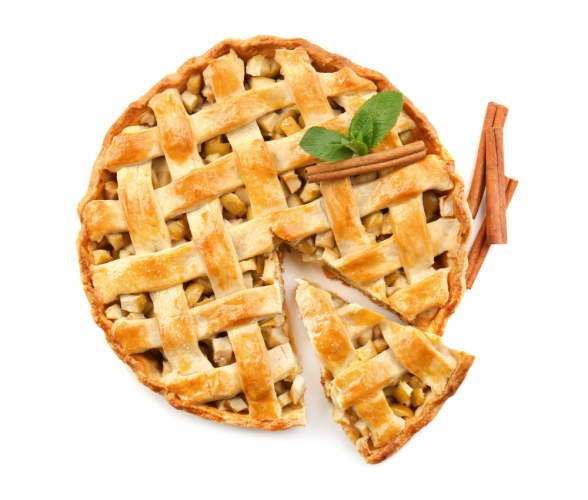The area of the large sector of this pie chart is 95 cm²

Find the angle at the centre of the large sector of the pie.

Use 3.14 for the value of Π

 86.0° 85.9° 83.2° 84.3° The angle at the centre of the sector is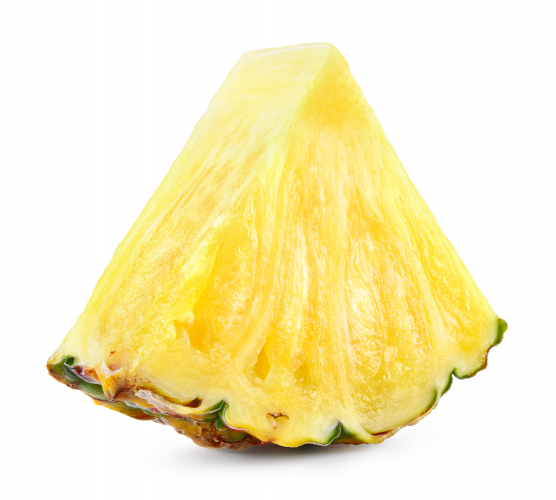The area of this sector of pineapple is 56 cm²

Find the angle at the centre of the sector of pineapple.

Use 3.14 for the value of ΠA sector of this cake has is going to be cut with an area of 85 cm²

Find the angle at the centre of the sector of cake once it has been cut.

Use 3.14 for the value of Π

152.3°

152.3°

152.3°

152.3°The pink sector of this pie chart has an area of 162 cm²

Find the angle at the centre of the sector.

Use 3.14 for the value of Π

152.3°

152.3°

152.3°

152.3°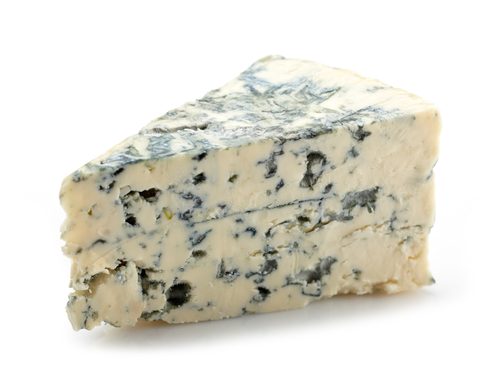The area of this sector of blue cheese is 15 cm²

Find the angle at the centre of the sector of cheese

Use 3.14 for the value of ΠThe area of the pie chart sector for nitrogen is 165 cm²

Find the angle at the centre of that sector of the pie chart.

Use 3.14 for the value of Π

 229.3° 259.6° 269.5° 295.6° The angle at the centre of the sector isThis fan forms a sector of a circle.

The area of the fan is 27 cm²

Find the angle at the centre of the fan

Use 3.14 for the value of Π

Match up

## Column B

Area of sector 32 cm², Radius 4 cm
229.3°
Area of sector 12 cm², Radius 4 cm
229.3°
Area of sector 25 cm², Radius 4 cm
179.1°
Area of sector 288 cm², Radius 12 cm
86°
• Question 1The area of this slice of pie is 20 cm&sup2;

Find the angle at the centre of the pie.

Use 3.14 for the value of &Pi;

63.7°
EDDIE SAYS
That pie looks tempting delightful, just like having a formula to help us. Formulas are delightful (the hard part sometimes is remembering them) If you apply the formula correctly you should get 20 x 360 = 7200 3.14 x 6 x 6 = 113.04 Now 7200 ÷ 113.04 = 63.7° (correct to 1 d.p)
• Question 2The area of the light blue part of this pie chart is 75 cm²

Find the angle at the centre of that sector of the pie chart.

Use 3.14 for the value of Π

 86.0° 85.9° 83.2° 84.3° The angle at the centre of the sector is
EDDIE SAYS
Calculators vary slightly. Make sure you know how to use yours correctly. Here I have calculated the two parts of the formula separately before dividing as my calculator doesn't like having all the information in one go. 75 x 360 = 27000 3.14 x 10 x 10 = 314 Now 27000 ÷ 314 = 85.98 (86.0° correct to 1 d.p)
• Question 3The area of the large sector of this pie chart is 95 cm²

Find the angle at the centre of the large sector of the pie.

Use 3.14 for the value of Π

EDDIE SAYS
Did you know that a slice of this pie costs \$5.50 in Jamaica and \$6.00 in Barbados. I know scandalous isn't it. That is the pie rates of the Caribbean for you. 95 x 360 = 34200 3.14 x 6 x 6 = 113.04 Now 34200 ÷ 113.04 = 302.5° (correct to 1 d.p)
• Question 4The area of this sector of pineapple is 56 cm²

Find the angle at the centre of the sector of pineapple.

Use 3.14 for the value of Π

25.7
EDDIE SAYS
Why do pineapples love extreme sports? Because they are hardcore. Are you getting familiar with the formula yet? I hope so just keep practicing. 56 x 360 = 20160 3.14 x 5 x 5 = 78.5 Now 20160 ÷ 78.5 = 25.7° (correct to 1 d.p)
• Question 5A sector of this cake has is going to be cut with an area of 85 cm²

Find the angle at the centre of the sector of cake once it has been cut.

Use 3.14 for the value of Π

152.3°
EDDIE SAYS
85 x 360 = 30600 3.14 x 8 x 8 = 200.96 Now 30600 ÷ 200.96 = 152.3° (correct to 1 d.p) Oh that is a nice size piece of cake to go with a cup of tea.
• Question 6The pink sector of this pie chart has an area of 162 cm²

Find the angle at the centre of the sector.

Use 3.14 for the value of Π

EDDIE SAYS
On questions such as this, they generally carry a lot of marks. Always show each stage of your working out to get as many marks as possible. 162 x 360 = 58320 3.14 x 12 x 12 = 452.16 58320 ÷ 452.16 = 129.0° (correct to 1 d.p)
• Question 7The area of this sector of blue cheese is 15 cm²

Find the angle at the centre of the sector of cheese

Use 3.14 for the value of Π

68.8
EDDIE SAYS
How do ducks eat cheese? On quackers. Lets hope that these activities are not driving you crackers. As long as you are consistent in your working out, you will find things will become easier. 15 x 360 = 5400 3.14 x 5 x 5 = 78.5 Now 5400 ÷ 78.5 = 68.8° (correct to 1 d.p)
• Question 8The area of the pie chart sector for nitrogen is 165 cm²

Find the angle at the centre of that sector of the pie chart.

Use 3.14 for the value of Π

 229.3° 259.6° 269.5° 295.6° The angle at the centre of the sector is
EDDIE SAYS
The same explanation again. That is the beauty of a lot of maths. You have a formula and just need to apply it 165 x 360 = 59400 3.14 x 8 x 8 = 200.96 Now 59400 ÷ 200.96 = 295.6.° (correct to 1 d.p)
• Question 9This fan forms a sector of a circle.

The area of the fan is 27 cm²

Find the angle at the centre of the fan

Use 3.14 for the value of Π

123.8
EDDIE SAYS
Are you a fan of these now? 27 x 360 = 9720 3.14 x 5 x 5 = 78.5 Now 9720 ÷ 78.5 = 123.8° (correct to 1 d.p)
• Question 10

Match up

## Column B

Area of sector 32 cm², Radiu...
229.3°
Area of sector 12 cm², Radiu...
86°
Area of sector 25 cm², Radiu...
179.1°
Area of sector 288 cm², Radi...
229.3°
EDDIE SAYS
I hope you are now applying the formula with ease. Like anything, it is easy once you know.
---- OR ----

Sign up for a £1 trial so you can track and measure your child's progress on this activity.

### What is EdPlace?

We're your National Curriculum aligned online education content provider helping each child succeed in English, maths and science from year 1 to GCSE. With an EdPlace account you’ll be able to track and measure progress, helping each child achieve their best. We build confidence and attainment by personalising each child’s learning at a level that suits them.

Get started